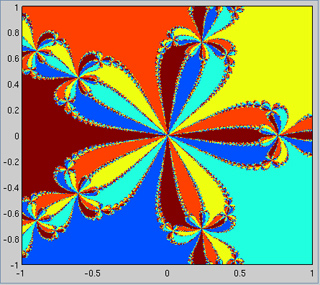18.S997 | Fall 2011 | Undergraduate
Introduction To MATLAB Programming
Vectorization and User-Defined Functions

## Complex Numbers

Complex numbers $$x+iy$$ can be dealt with “natively” in MATLAB®. This means that you can take powers and roots of any number. One surprising and very powerful fact about complex functions is that a single derivative (not a $$2\times2$$ Jacobian) gives you the full derivative information (assuming that the function is analytic).

Exercise 12: Modify your 1D Newton’s method to find the basins of attraction of a interesting (degree > 3) polynomial. Plot the basin of attraction as before, but now you will have to create your initial value by $$z=x+iy$$. For the roots: either use a polynomial for which you know the roots, or find the roots in advance, by using your own Newton solver.

Here’s the basin of attraction of the roots of $$z^5+1$$:A plot of the basin of attraction with complex roots.

How long does it take your code to make a 1000 by 1000 plot (that is, a plot in which both the x- and y-axes have 1000 points)? If it’s more than 1 minute, your code is too slow. The reason for that is that MATLAB can be inefficient with explicit loops. In this case, we have nested loops and a plot command inside them; this is a disaster!

We need to “vectorize” the calculation and only plot once at the end after we have the result. This means instead of having MATLAB loop over the points and calculating each one at a time, you set them all up in a big matrix/vector and iterate on all of them together:

We let a matrix $$A$$ hold all the values of the iterations that correspond to the different points we will end up plotting. Thus we can set up the initial $$A$$ as follows:

N=1000;
x=linspace(-5,5,N);% linspace is another function that creates vectors.
A=ones(N,1)*x + 1i*y'*ones(1,N);


Exercise 13. Make sure you understand:

• What does ones do? Find out using help ones
• Can you guess what zeros does?
• We can also use meshgrid for this, can you understand how to do it?

In the one-at-a-time Newton’s method the update step is

\begin{equation} \label{eq:7} x_{n+1}=x_n-\frac{f(x)}{f’(x)} \end{equation}

We want to do the same only updating all of $$A$$ at every step. It would work just fine if we could write

A=A-f(A)./f'(A); %Notice the ./ ? This means, POINT-WISE multiplication,
% not linear algebra


And we can. We only need to make sure that we define functions $$f$$ and $$f’$$ that know how to work with a matrix and return the right answer. We can also make our code more flexible by using our own functions ($$f(x)$$ and $$f’(x))$$. Here is how to define simple (one command) functions:

f=@(x) x.^5+1; %Notice the point here? However there's no such thing as .+
fp=@(x) 5*x.^4;


After defining f and fp, they can be used like any other MATLAB function:

>> f(1)
ans =
2
>> f([1 2 3])
ans =
2    33   244
>> f([1 2; 3 4])
ans =
2          33
244        1025


Last, but not least, plotting a 2D surface (the following code has nothing to do with our problem, but it illustrates how to plot a nice 2D surface):

x=linspace(0,2*pi);
y=x';
[X,Y]=meshgrid(x,y);%x,y are vectors,X,Y are matrices
Z=sin(X).*cos(Y.^2);
pcolor(X,Y,Z);


There are slight variations to pcolor: mesh, surf, and more. Learn about them using the help command.

Project 2. Update your Newton’s basin of attraction code to work using matrix-at-a-time update. Use pcolor to create the resulting basin plot. Your result should be much faster.